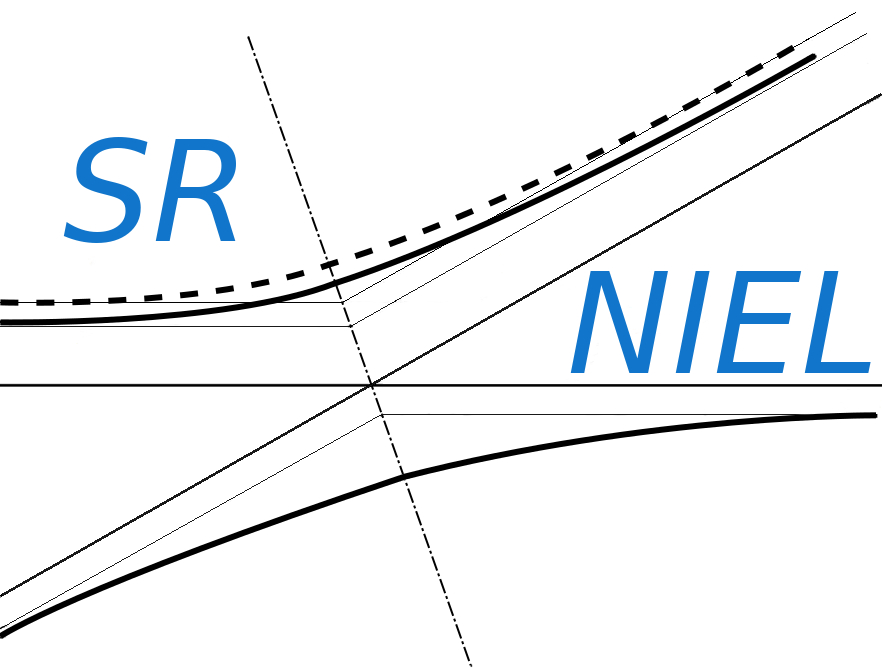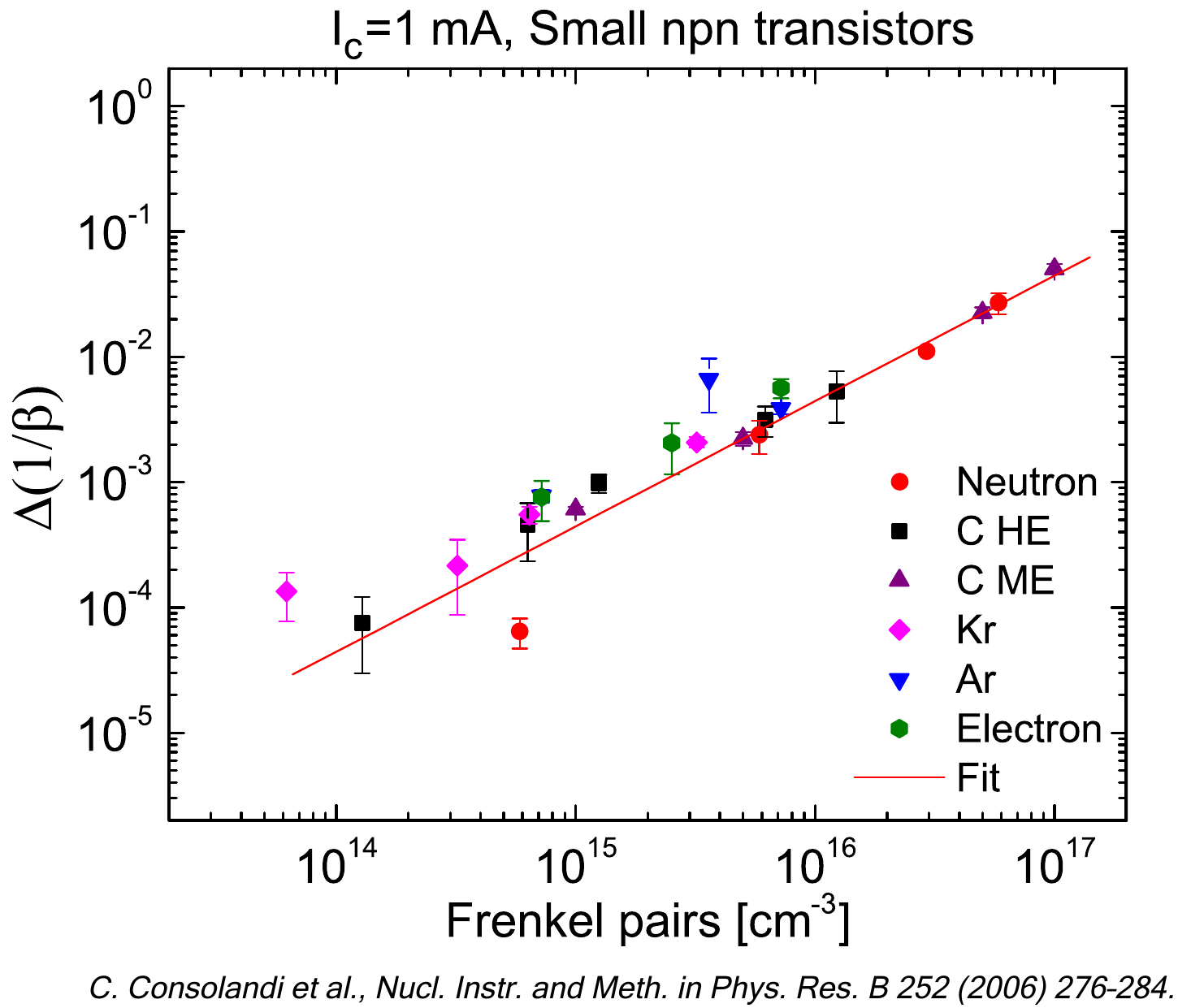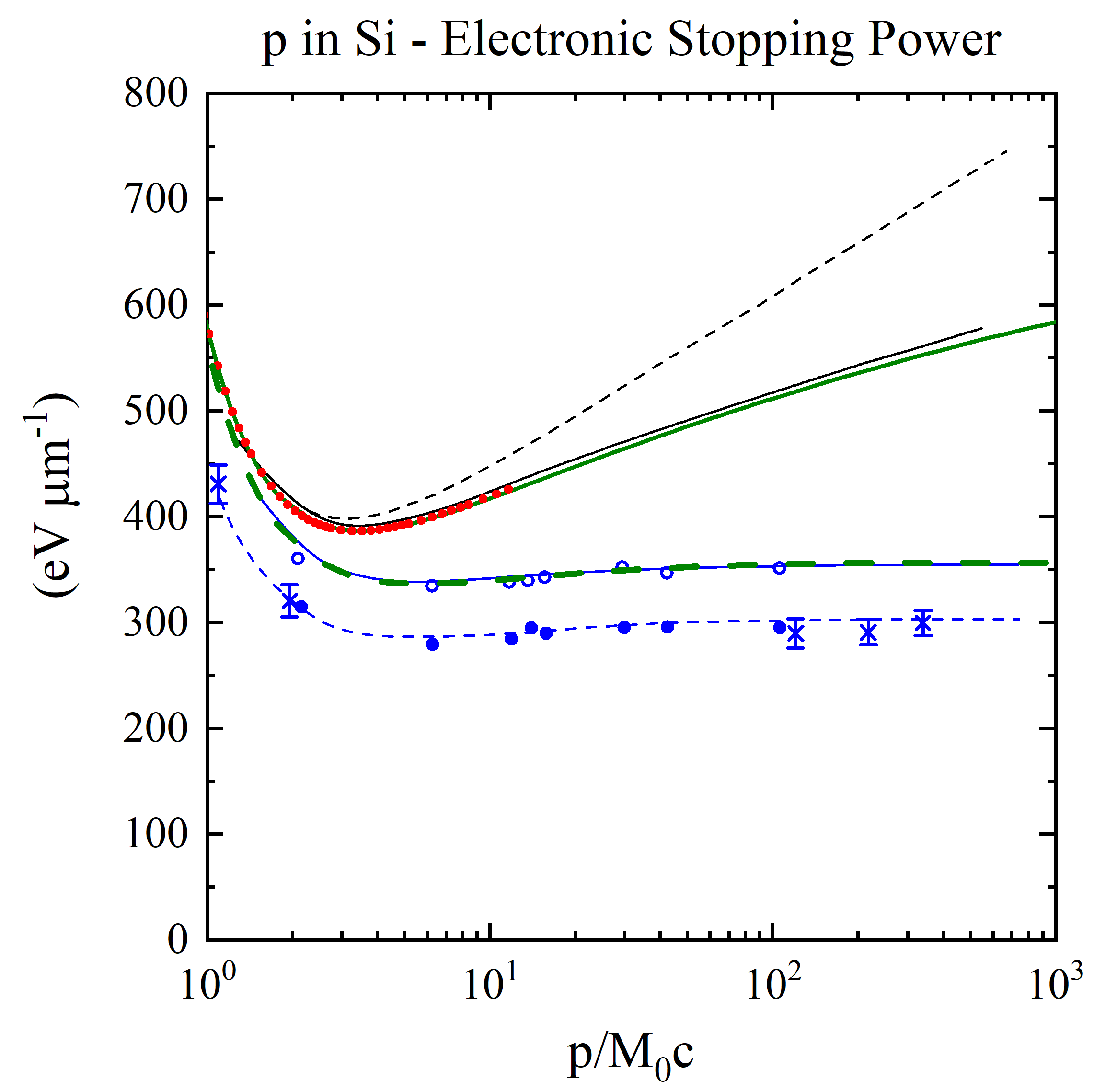SR-NIEL – 7 Screened Relativistic (SR) Treatment for NIEL Dose Nuclear and Electronic Stopping Power Calculator (version 8.0)The current web calculator regards the restricted energy loss implemented within SR-Framework (see Restricted energy-loss treatment within SR-NIEL framework).

The following link allows one to access to the Restricted energy-loss calculator and ionizing dose with extension to high energies for spectral fluence of protons and ions:

### How to use this restricted energy-loss calculator and ionizing dose with extension to high energies for spectral fluence of protons and ions

This tool calculates the Restricted Energy Loss for a for a spectral fluence incident on a material.

The input parameters and options for the tool are described below. When the input form has been completed, pressing the "CALCULATE" button will start the calculation and open the "Results" page (allow for pop-up in your browser settings). The result page will be also linked at the bottom of the calculator page.

The physical mechanism of ionization loss by collisions, energetic electrons - the so called δ-rays - can result from the collision process. However, we recall to the user that, as the incoming particle energy increases, high-energy δ-rays generated in the process of collision energy losses can escape from the absorber (e.g., see webpage). Thus, the energy lost by the incoming particle differs from that deposited in the absorber which approches an approximate constant value at high energy (the so-called Fermi plateau). This is illustrated, as an example, in the following figure (Figure 1) in which the calculated stopping power and measured deposited energy of massive particles with z=1 in silicon are shown for 1< <1000. = 11.61 is that for a proton with kinetic energy of 10 GeV.

In the Restricted energy-loss treatment within SR-NIEL framework and in the corresponding estimate of ionizing dose, the physical mechanism of energy deposition per unit length in a medium (thus, the resulting computed value of restricted energy loss) depends on the effective detectable maximum transferred energy (W0) and, in turn, on the overall traversed path to which W0 is related.

For the validity of the implemented restricted energy‐loss formula [Eq. (2.29) at page 70 of Leroy and Rancoita (2016)], the condition is that the W0 is large enough in order to neglect the shell binding energies of atomic electrons. In the present web calculator, W0 is set to be larger than five times the ionization energy of the absorber.Figure 1. Energy loss in silicon (in units of eV/μm) versus βγ (= p/M0c, where M0 and p are the rest mass and the momentum of the incoming particle with z=1) (see also [Rancoita (1984)]). From the top, the first two curves are: the stopping power without (broken curve) and with (full curve) the density-effect correction (e.g., see Chapter 2 of [Leroy and Rancoita (2016)]) . The red points for incoming protons up to 10 GeV (βγ =11.61) are obtained from the electronic stopping power SRIM calculator currently implemented in this website. The solid green curve for incoming protons was obtained from the SR-framework electronic stopping implemented in this website: for proton energies larger than about 16.7 MeV it agrees agrees with SRIM within about 0.5% (see Figure 6 here). For protons with energy of about 510 GeV (βγ around 550), the difference between the electronic stopping power from SR-framework and that from Esbensen calculation (black solid curve) is 1.86%. The dashed green curve was obtained from the present SR-framework restricted energy loss with W0=500 keV (in agreement with the value discussed at page 73 of ). The following two other curves - compared to experimental data for detector thicknesses of 300 (× from [Hancock, James, Movchet, Rancoita and Van Rossum (1983)]) and 900 μm ◦ and from [Esbensen et al. (1978)]) - are, respectively, i ) the top one (solid line in blue) for the restricted energy-loss with the density-effect taken into account and ii) the bottom one (dashed line in blue) for prediction of the most probable energy-loss.

As discussed at page 70 of [Leroy and Rancoita (2016)] for all incoming particle energy for which the maximum transferred energy W (see Eq. (1.29) of [Leroy and Rancoita (2016)]) is lower than or equal to W0 there is no difference in between the SR-Framework electronic stopping power and the restricted energy loss (see Figure 2).Figure 2. Energy limit (in MeV/amu) below which there is no difference in between the SR-Framework electronic stopping power and the restricted energy loss as a function of W0.

### Input Parameters:

- Incident particle (for Protons&Ions Calculator)
- Target material
Effective detectable energy W0
- Spectral fluence

### Incident Particle

In the Protons&Ions Calculator, using the pull down menu, the user can select the species of the incident particle, either a proton or one of the elemental ions.
Except for proton and alpha particle masses, the user can also modify the mass (in amu) of the incident particle (e.g., for all isotopes one can refer to this page): the default mass is that of the most abundant isotope (MAI). Further information are available at the following webpage

### Target Material

In the section "Target Selection" it is possible to specify an User Defined target material or a predefined Compound material. User can also select the target as gas, this is allowed only for single element and natural gas target (H, He, N, O, F, Ne, Cl, Ar, Kr, Xe, Rn).

The stopping power in target gases is usually higher than that in an equivalent solid target. The Gas/ Solid correction disappears for higher velocity ions with energies above 2 MeV/amu. But at lower velocities the effect can be quite large - almost a 2 times change in stopping bacause of the Phase effect near the Bohr velocity, 25 keV/amu.

in the User Defined section individual elements can be selected as well as the composition of the target material choosing the number of elements in the compound. The required parameters for each element are:

- Atomic number (Z)/Chemical symbol
- Stoichiometric index or element fraction

Electronic Stopping Power for User Defined Compounds can be determined by means of Bragg's rule, i.e., the overall Electronic Stopping Power in units of MeV cm2/g (i.e., the mass electronic stopping power) is obtained as a weighted sum in which each material contributes proportionally to the fraction of its atomic weight. For instance, in case of a GaAs medium ones obtains (e.g., Eq. (2.20) at page 15 in [ICRUM (1993)]):whereand AGa [AAs] are the Electronic Stopping Power (in units of MeV cm2/g) and the atomic weight of Gallium [Arsenic], respectively.

For few compounds belonging to the ICRU list the parameters employed for the energy loss formula (including those for the densiity effect) are reported in Table II of Sternheimer et al. (1984).
Further discussion can be found in the help of the SR-framewrok electronic stopping (see also results page).

### Spectral Fluence

This section define the points of the spectral fluence as a function of energy.
The input format is one point per line (Energy - Flux , separated by a space or tab); it is also possible to copy and paste values. The minimum value of the particle spectral fluence is 1 eV.

### Result

The result page contains the input spectral fluence and the Restricted Energy Loss curve in MeV cm2/g, calculated at the same energies defined in the spectral fluence. For every energy points the restricted energy loss per unit area and energy is calculated and displayed in the second graph. The mass restricted energy loss per unit area and energy is integrated at the energy steps defined in the spectral fluence and the results of every single bin is given in the last column of the table (restricted energy loss per unit area).